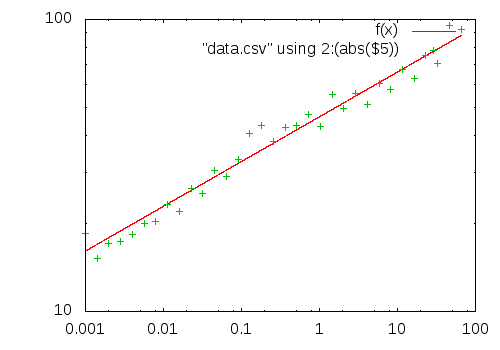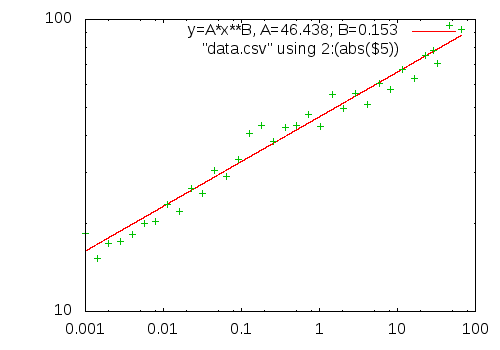# Uellue's Blog

## Include a numeric variable in a Gnuplot title or legend

I was fitting a function to some data using Gnuplot, and then I wanted to include the result of the fit in the legend of my plot. Well, up to here the script looks like the following:

``````# my function to fit the data
f(x) = A*x**B

# initial values for the fit
A = 1
B = 1

# do the fit, use column 2 as x and the absolute value of column 5 as y
fit f(x) "data.csv" using 2:(abs(\$5)) via A, B```
[... lots of output from fit ...]
```

Now the variables A and B have a new value, resulting from the fit:

````print A, B`
46.437770413374 0.153260265112221```

I can plot the data together with the fit:

``````set output "data.png"
set terminal png

set logscale xy
set samples 10000

plot f(x), "data.csv" using 2:(abs(\$5))``````

This generates the following image:Now I would like to have a legend for the fit like the following:

`"y=A*x**B, A=46.437; B=0.153"`

After digging a bit in the documentation, I discovered that Gnuplot has an `sprintf` function that can be used more or less everywhere where a string literal would work. So that's how I got what I wanted:

``````[...]
plot f(x) title sprintf("y=A*x**B, A=%.3f; B=%.3f", A, B) ,\
"data.csv" using 2:(abs(\$5))``````

And that's what it looks like:Well, in the next steps the legend could be moved to the lower right corner, but I'll leave that as an exercise for the reader ;-)

Dear Ulle,